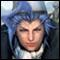Math Is Fun Forum

Discussion about math, puzzles, games and fun.   Useful symbols: ÷ × ½ √ ∞ ≠ ≤ ≥ ≈ ⇒ ± ∈ Δ θ ∴ ∑ ∫  π  -¹ ² ³ °

You are not logged in.

#1 2005-08-26 01:47:40

ahgua
MemberRegistered: 2005-08-24
Posts: 25

Strange formula

There is a formula to find area of any polygon drawn on a graph.

Suppose the vertices of the polygon are (x1,y1) ; (x2,y2) ; (x3,y3) ; (x4,y4).

Area of the polygon on the graph = 1/2 x | x1y2 + x2y3 + x3y4 + x4y1 - x2y1 - x3y2 - x4y3 - x1y4 |

| | is the absolute sign.

How do you prove that this formula is true?

P.S. The textbook didn't teach me this!

Last edited by ahgua (2005-08-26 03:45:27)

Life is a passing dream, but the death that follows is eternal...

Offline

#2 2005-08-26 10:28:24

MathsIsFunRegistered: 2005-01-21
Posts: 7,710

Re: Strange formula

I wrote a program to do this once.

Draw the polygon on a sheet of graph paper.

Pick the first line and work out the area from that line down to the x axis (it is just the average of the heights times the x-difference), at this stage it helps to sketch this!

Now do the same for the second line and proceed around the polygon.

Then just add up the areas! (Note: some areas will be negative, as I said, it helps to sketch it.)

So the area would be:

(x2-x1)*(y1+y2)/2
(x3-x2)*(y2+y3)/2 +
(x4-x3)*(y3+y4)/2 +
(x1-x4)*(y4+y1)/2 +

(This might end up the negative of the area, so the || can be used later)

Anyway, if you rearrange that, you might get your product formula.

"The physicists defer only to mathematicians, and the mathematicians defer only to God ..."  - Leon M. Lederman

Offline

#3 2005-08-27 22:09:19

wcy
Member
Registered: 2005-08-04
Posts: 117

Re: Strange formula

i think it can be proven by dividing the polygon into many smaller polygons, eg triangles

Offline